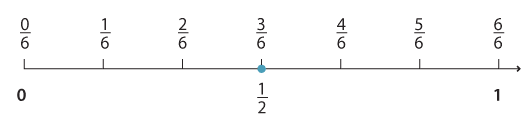### Equivalent fractions

Equivalent fractions are located on the same place on the number line.

The number line shows that $$\dfrac{3}{6} \text{and} \dfrac{1}{2}$$ mark the same place. That is, $$\dfrac{3}{6} \text{and} \dfrac{1}{2}$$ are equivalent fractions.Detailed description